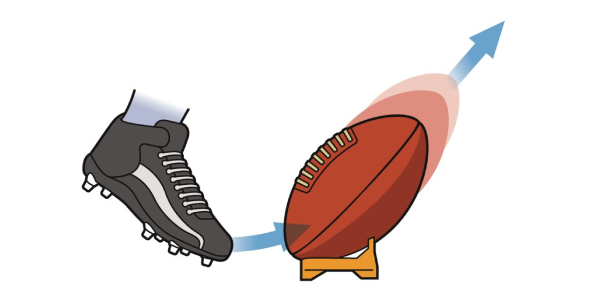# Quiz On Forces: Trivia!

7 Questions | Attempts: 3437Settings.

• 1.
Which of these is the best way to measure a force?
• A.

Using a force meter

• B.

Using a thermometer

• C.

Using a ruler

• 2.
Forces can be
• A.

Pushes only

• B.

Pulls only

• C.

Pushes and pulls

• 3.
What is the unit of force called?
• A.

The newton, n

• B.

The neutron, N

• C.

The newton, N

• 4.
Why does an astronaut weigh less on the Moon than on the Earth?
• A.

The moon has no atmosphere

• B.

The moon has no gravity

• C.

The force of gravity is weaker on the surface of the Moon than on the surface of the Earth.

• 5.
What is the weight of a 20 kg box on the Earth?
• A.

20N

• B.

2N

• C.

200N

• 6.
What can we say about the forces on an object that is not moving?
• A.

There must only be one force acting on it

• B.

The forces must be balanced

• C.

It must be very heavy

• 7.
One way to increase the turning force on a spanner is to:
• A.

Use a shorter spanner

• B.

Use a longer pivot

• C.

Use a longer spanner

## Related TopicsBack to top
×

Wait!
Here's an interesting quiz for you.